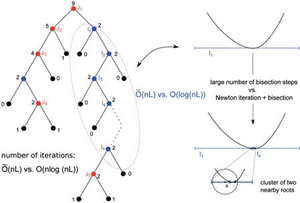# Exact Solving of Polynomial Systems and Applications in Geometric Computing

## Exact Solving of Polynomial Systems and Applications in Geometric Computing

Solving systems of polynomial equations poses a fundamental problem of algorithmic mathematics. For m given polynomials in n variables, all points in n-dimensional space should be found such that all of the polynomials simultaneously take on the value 0. Such systems of equations occur in nearly all areas of mathematics, physics, and engineering. The research group “Computer Algebra and Geometric Computing” primarily investigates such systems as they typically arise from geometric problems. Most algorithms from computational geometry or computer-aided design require such exact calculations with geometric objects. For example, one might wish to find the intersection points of two curves, or to determine whether multiple curves intersect at the same point. Such problems normally lead to a low-dimensional system of equations, in which some of the polynomials may be of high degree. A common solution method from computer algebra is the implicit projection of all of the solutions onto a line. By using algebraic transformations, one then fi nds a so-called elimination polynomial, whose zeroes are exactly the projections of the solutions of the system. In many cases, the general problem can thus be reduced to the special case m=n=1.

In comparison to pure numeric methods, as are almost exclusively applied in industrial software packages, the above-described approach allows the development of complete and exact methods. This means that even for particularly difficult inputs, all solutions are found and are provable as such. Unfortunately, there are also downsides: the symbolic transformations generally lead to a very high computational demand, even if the solutions are of a relatively simple geometric nature (for example, solutions that are simple or well separated from one another). This maladaptive behavior results from the fact that the underlying algebra is “ignorant” with respect to such simpler situations. A further problem of the projection approach is that the corresponding elimination polynomial exhibits high complexity, which makes finding its zeroes difficult.

In previous years, the research group has developed methods to considerably improve the efficiency of elimination procedures. An essential goal is always to replace unnecessary symbolic computations with certifi ed numeric ones, however, without sacrificing exactness or completeness. In addition, we develop efficient methods to carry out the remaining, and also necessary, symbolic steps. For the computation of the elimination polynomial for bivariate systems, as well as that of the greatest common divisor of polynomials, we have developed a new algorithm, whose implementation on graphics hardware takes greatest advantage of the parallelizability of the method. In comparison to the fastest competing implementations, the aforementioned computations can thus be speeded up by a hundredfold.

Through the combination of results from different areas, such as computer algebra, numerical mathematics and algebraic geometry, we have also succeeded in developing procedures that require only those symbolic calculations which can be delegated to graphics hardware.

Thus, with regard to speed, we were able to catch up to numerical methods – which deliver no additional guarantees – for the fi rst time. In parallel, we also were able to prove the theoretical efficiency of our algorithms. The corresponding bounds on the number of steps needed to fi nd the solution improve on the best previously known bounds by multiple orders of magnitude. Based on present knowledge, there is evidence that these are actually nearly optimal.##### Combination of the Descartes method (linear convergence) and Newton‘s iteration (quadratic convergence) produces a simple and nearly optimal process for the determination of the real zeroes of a polynomial.

We also succeeded in finding a similar breakthrough for the classical special case where m = n = 1. In the 80s and 90s, complex algorithms were first developed to find the zeroes of a polynomial in nearly optimal (theoretical) runtime. However, the practical relevance of these procedures has so far not been confirmed. Taking a different approach, we were able to modify a simple, and in practice very efficient, process such that it could compete with the optimal methods, even in terms of theoretical complexity.

## Michael SagraloffDEPT. 1 Algorithms and ComplexityPhone +49 681 9325-1006Email msagralo@mpi-inf.mpg.deInternet www.mpi-inf.mpg.de/departments/d1/areas/software.html